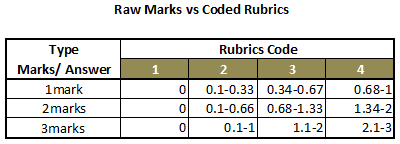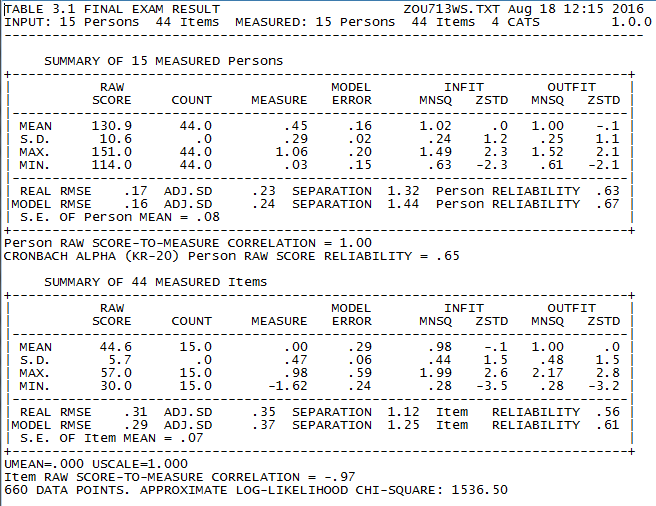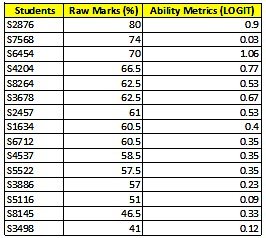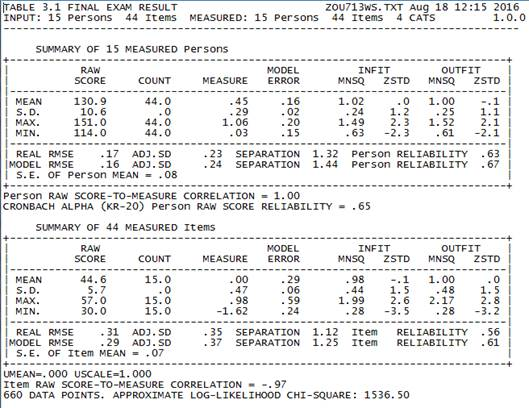# Measurement of Rubrical Essay-based Test Using Rasch Model

## Abstract

There are many educators use raw score as measurement for student’s ability, but it never truly measures the right measurement. The raw score should be converted into a right linear metrics for ability measurement. This study is to implement rubrical analysis or assessment for essay-based test using Rasch Model (RM). It is to produce a reliable and accurate measurement procedure for student’s performance in essay-based test. The initial study has identified the concept of RM to be applied in educational test or examination. The design and development stages has produced a comprehensive but simplified procedure template of scientific analysis within Bond&Bond Steps software. The proposed procedures contains of measuring score of accurate student’s ability in LOGIT unit, providing of student’s result profiling, and measuring reliability of the test set and the student’s answers,. The procedure is designed for essay-based test which is more difficult to be analysed, compared to the multiple choice type. This procedure converts the student’s answer into rubrical ratio-based scale to be most accurately measured and definitely better than the common practice of merely analysis on raw marks for each question. It will show true student’s performance of cognitive performance (test) which represents the true student’s ability, in order to accurately measure the right outcome.

Keywords: Student’s performancelearning assessmentrubrical analysisrasch measurement model

## Introduction

Measurement is fundamental in education. A comprehensive and effective education contains of good content, wise objective, positive outcomes, effective instructions and right assessment with fair and accurate measurement. Rasch measurement model (RMM) was accepted worldwide as better method to verify the validity of measurement construct (test question construct) and accurate measure of student’s ability in logit (log odd unit) scale. Lately, many researchers are aware of the reliability of RMM to be applied in social sciences study, especially on psychometric measurement, but not many teachers, lecturers or educators use RMM in educational assessment. This procedure will help much educators, to precisely and accurately measure the ability of students within simplified steps. This model is in parallel with aspiration of national educational policy which stresses much on outcome-based education. This has produced a comprehensive templates and procedures of analysis for lecturers/ teachers/ educators to use as basic reliable assessment. The procedure will engage with Bond&Fox Steps software to transform ordinal data (answer’s score/ marks) into equal interval scale (probability of student’s ability) which is more precise and accurate in psychometrical result, rather than normal result with calculation of raw score.

This should be used for accurate student's performance as better alternative for the current practice which counts on merely the raw score of question marks. This procedure provides linear metrics of ratio-based measurement in LOGIT, as practiced in most developed countries. Most practices in our current examination use essay-based approach and this rubrical analysis is the most compatible solution. This simplified procedure is easy and friendly for all lecturers without any necessary of understanding Rasch statistical analysis or Bond&Fox Steps software in depth.

## Problem Statement and Objective of the Study

In educational assessment, there are still many educators uses raw score as measurement for student’s ability, but raw scores never been true measure (Azrilah, 2012; 2008; Bond, 2007; Wright, 1989). Not all educators are proper researchers. Therefore, there is a crucial need to provide a simplified model as a bridge between accurate scientific analysis in research and raw educational assessment, as well as to upgrade the quality of student’s ability assessment to be more accurate and reliable. In addition, it is difficult to analyse the student’s response especially in essay-based test. This simplified procedure proposes the method of rubrical analysis as student’s responses.

The research questions are:

• How to analyse student's responses in essay-based test using Rasch Measurement Model?

• How to produce reliable and accurate student's performance in essay-based assessment?

The research objectives are:

• To implement rubrical analysis or assessment for essay-based test using Rasch Model.

• To produce a reliable and accurate measurement procedure for essay-based student's performance.

## Methodology

As common practice in education, test is administered among the students, to sit normal examination process. Student’s answers then will be keyed in to Microsoft Excel format, in which lecturers are very familiar. The next step is to convert the student’s answers (data) into the Bond&Fox Steps software for reliability analysis. The data in Microsoft Excel’s format needs to be resaved in .prn format, to allow them to be opened using Bond & Fox Steps for more friendly use in educational purposes. This study has determined a class of 15 students from Environmental Technology programme (EVT229), from the Faculty of Applied Science, Universiti Teknologi Mara Malaysia.

The rest is only to extract person measure and item measure by only one click within Steps software. This can automatically identify and sort good students with the highest score to weak students with the lowest score. These simple steps may be used as tools for accurate assessment of reliable student’s ability or performance in such course in a linear ruler with LOGIT measure. This procedure may allow us to easily understand and utilize in any courses or programs which aim for learning outcome of the course as reliable student’s performance.

The study used the Final Examination set of Environmental Ethics course (IPK661) for June 2016 examination. The set has one question with four sub questions, and three questions with three sub questions. Each sub question carries one, or two or three marks provided for each answer. Using analysis function in the software, the result of item and student’s reliability is also can be shown. This also can determine the reliability of the overall student’s answers and detect any problems appeared in detailed result of individual analysis. The observed variance and the error variance values will be taken into calculation to find reliability level, as shown below:

(Observed Variance – Error Variance) / Observed Variance = Reliability Score

This study uses common statistical measurement where it accepted the range of reliability score between 0.5 to 1.0 as reliable and acceptable. It is similar to Cronbach Alpha value which accepts the value between 0.5-1.0. However, in the real practice as formal assessment towards students, it is recommended to accept only excellent reliability of student’s answer to be more valid and highly accepted which is between 0.8 and 1.0 only. This is more equivalent to common measurement scheme in education which is between 80% - 100% to be considered A. Otherwise, the students will be required to retake the test, or it would be recommended to investigate the real factors behind of the inconsistency of the responses.

## Why Rasch Model?

The use of Rasch Measurement Model (RMM) is based on the several distinguished reasons, as follows:

• Rasch offers a new paradigm in education longitudinal research.

• Rasch is a probabilistic model that offers a better method of measurement construct, hence a scale.

• Rasch gives the maximum likelihood estimate (MLE) of an event outcome.

• Rasch reads the pattern of an event thus predictive in nature which ability resolves the problem of missing data. Hence, it is more accurate.

• Rasch transforms ordinal data into equal interval scale, which is more appropriate for humanities and social science research.

• Rasch measures item and task difficulties, separately and accurately (Mohd Nor Mamat, 2012).

2.2 Procedure of Student’s Ability Measurement

A comprehensive procedure of measurement could be simplified as follows:

• Students’ answers scripts will be marked as usual

• Students’ raw score will be recoded to rubrical score for analysis

• Data will be analysed using Bond&Fox Steps software

• Results of students’ accurate performance score (LOGIT) will be delivered

• Students’ profiling, reliability score and test’s reliability score will be produced

This simplified procedure needs only few simple steps to be applied. For the first steps, after the answer’s script has been marked (as usual practice), it need to be recoded into rubrics scale, like Likert scale. As for IPK661 question set has different values for each answer, it is necessary to recode accordingly.Figure 1: Fig. 1. Raw marks vs coded rubrics.

The rubrical value would be keyed into EXCEL format and saved as .prn format. The .prn file would be opened using Bond&Fox Steps and few clicks, resulted the display of reliability score for the question set and student’s answer which is acceptably reliable (i r =0.56) and (p r =0.63) with Cronbach alpha value at 0.65. In the future practice, this result will help much for teachers and educators to evaluate the quality of test questions before distributing to the students.Figure 2: Fig. 2. Summary of reliability and Cronbach alpha values.

The last procedure in this study is to analyse the rubrical values of student’s answers to be in a linear metrics, LOGIT unit. With one more click, we have the result of students (in rank order) within log odd unit (LOGIT) or probabilistic ratio-based measure. Any kind of human ability measure should be assessed using this kind of measurement, not merely calculating the marks given to each question. In this case, the more comprehensive analysis may be made to identify unique problems of every single student. This procedure would identify the right measure of student’s ability and put them in rank order.

2.3 Result of Student’s Ability

Among 15 students involved, 13 passed the examination with score of between 51-80 marks, while 2 of them failed with 41 and 46.5 marks. After all these marks were converted into LOGIT unit, the result showed that all students have positive result, but only six of them have passed over mean value (0.43) which means above 50%. Student’s ability score are between 0.03 to 1.06 logit. It could be concluded that the use of raw marks for every question does not show the real values of student’s ability. From the map below, we could conclude student with ID S6454 is excellent and able to answer all questions, and all students could answer the mean level of questions.

From the above analysis, all students are fit with the model, which means those students are normal and their answers are reliable. The rank of student’s ability is shown clearly, in which the student with ID S6454 scores 1.06 logit to be the most capable students in answering questions, while the student with ID S7566 scores the lowest value, which is 0.03 logit.Figure 4: Fig. 4. Raw marks vs ability metrics score.

Using this procedure, students would be assessed according to their ability, not merely on counting raw marks provided for each question. After the analysis, it was found that the best student with raw marks (80) is not the best able student in logit (0.9). Similar to that finding, the weakest student with raw marks (41) is not the least able student in logit (0.12). The most able student (1.06) is the student who got 70 marks, who is the third at the rank, while the second best student with raw marks (74) is actually the least able students in logit. For further study, it is an interesting study to find correlation between student’s marks in the final examination and student’s ability performance using logit unit.

2.4 Reliability of Test and Student’s Answer

Face validity essentially looks at whether the scale appears to be a good measure of the construct "on its face". While construct validity is referring the analysis or outcome of the theories and ideas on the study being carried out. The actual instrument construct that is developed should reflect the theories initiated or chapters taught, in case of assessing educational course outcomes. For the face validity, the committee endorsed the question set as the valid and reliable instrument for the course assessment. Reliability wise, it was agreed that the use of such instrument would lead the way to understand the course accordingly. In educational practice, students are respondents and their answers could be analysed for the reliability of test questions or instrument’s construct.Figure 5: Fig. 5. Reliability of questions and studens.

In this study, the analysis showed that the question set has low reliability which is 0.56 value, while student’s answer has low reliability value at 0.63 score. The Cronbach Alpha value also showed that the test reliability is low at 0.65 score. This means, the examination question, prepared by the university is low reliable and the student’s answer is also low reliable, but still accepted. In our current practice, this reliability of question paper and student’s answers are seldom checked or analysed, made our examination remains untested and possibly unfair for the students.

Instead of verifying validity of the examination paper, this procedure with Rasch measurement model can also recognize true normal good students or students with problems, or abnormal (unique) students, individually. This is very important to be observed and wisely taken into consideration, as the assessment is done onto human ability. From the above analysis, all students are fit with the model, which means those students are normal and their answers are reliable.

## Conclusion

A well scientifically reliable-proven question set of examination will give us valid data of student’s ability and performance in any course for the meaningful accurate assessment. Rasch offers a mean of verifying question item, subsequently the validity of the question set as well as student’s answers reliability. The certainty of an instrument to measure outcomes of the teaching and learning, which is student’s ability of answering the questions of what has been learned is now scientifically vetted, tested and validated, and more important is reliable for the purpose. Logit unit, the probabilistic ratio-based measure scale is more accurate for human performance assessment. In additional, this esay and simplified procedure would be the best solution to be implemented by all educators without knowing statistical elements in detail.

## Acknowledgment

Acknowledgment to Universiti Teknologi Mara Malaysia for funding this research and publication.

## References

1. Azrilah Abdul Aziz et al (2012). Asas Model Rasch: Pembentukan Skala dan Struktur Pengukuran. Bangi: Universiti Kebangsaan Malaysia Press.
2. Azrilah Abd Aziz (2008). “Developing an Instrument Construct Made Simple”: Winstep and Rasch Model Workshop. Shah Alam: Universiti Teknologi Mara 30-31 December 2008.
3. Bond, Trevor G. & Fox, Christine M. (2007). Applying the Rasch Model: Fundamental Measurement in The Human Sciences. New York: Routledge.
4. Bond, Trevor G. and Fox, Christine M. (2007). Applying the Rasch Model (Second Edition). New Jersey: Lawrence Erlbaum Associates Publishers.
5. Fisher, William P. Jr (2007). Rasch Measurement Transactions (http://www.rasch.org/rmt).
6. Linacre, J. M & wright, B. D. (2000). Winsteps: A Rasch Computer Program. Chicago: MESA Press.
7. Mohd Nor Mamat (2012). Development of Hadhari Environmental Ethics Among Environmental Ethics Student at Tertiary Education. Shah Alam: PhD Thesis (unpublished)
8. Wright B. & Linacre J. (1992). “Combining and splitting categories”: Rasch Measurement transactions 6: 233-235.

22 November 2016

#### Article Doi

https://doi.org/10.15405/epsbs.2016.11.80

#### eBook ISBN

978-1-80296-015-0

16

-

1st Edition

1-919

#### Subjects

Education, educational psychology, counselling psychology